# Objectives Use long division to divide polynomials Use

• Slides: 30Objectives § § Use long division to divide polynomials Use synthetic division to divide polynomials Evaluate a polynomial using the Remainder Theorem Use the Factor Theorem to solve a polynomial equation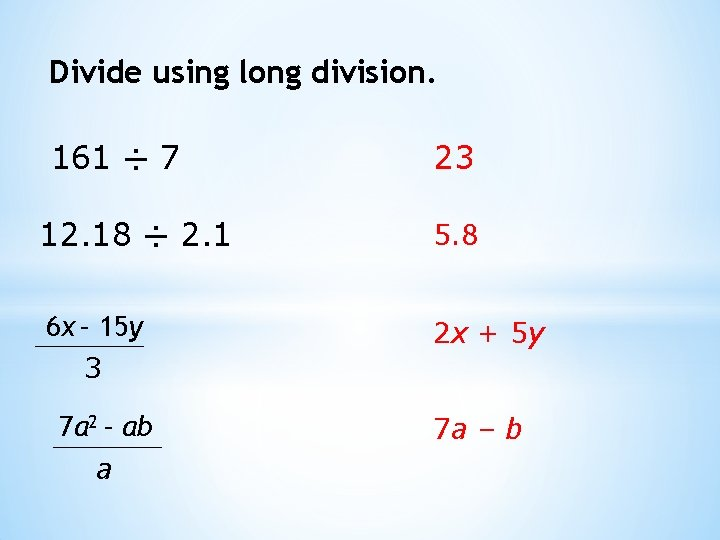Divide using long division. 161 ÷ 7 23 12. 18 ÷ 2. 1 5. 8 6 x – 15 y 3 2 x + 5 y 7 a 2 – ab a 7 a – b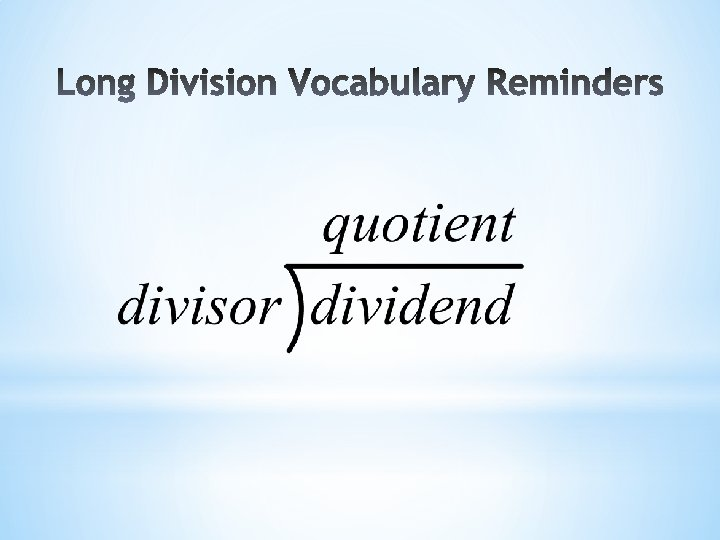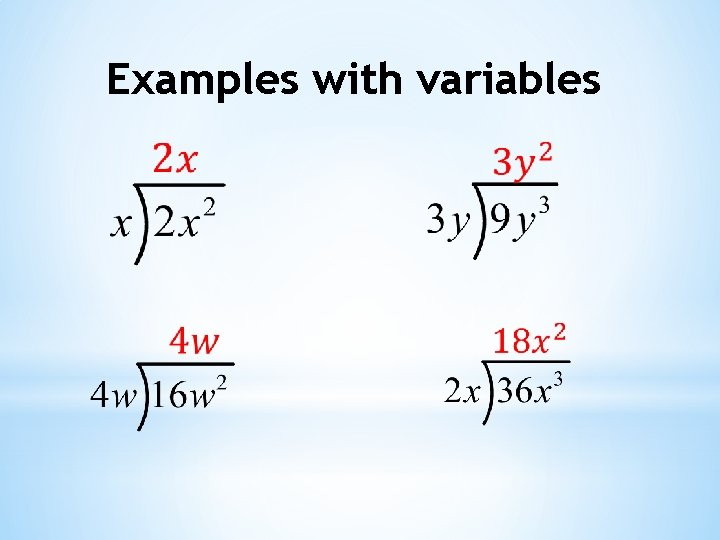Examples with variables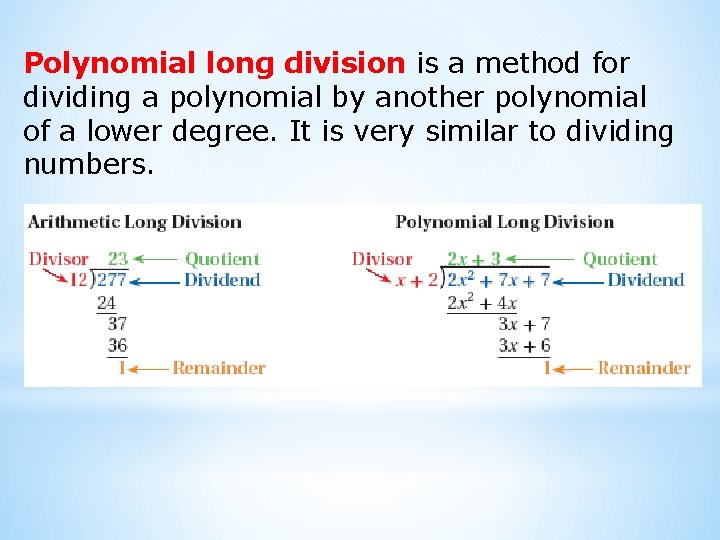Polynomial long division is a method for dividing a polynomial by another polynomial of a lower degree. It is very similar to dividing numbers.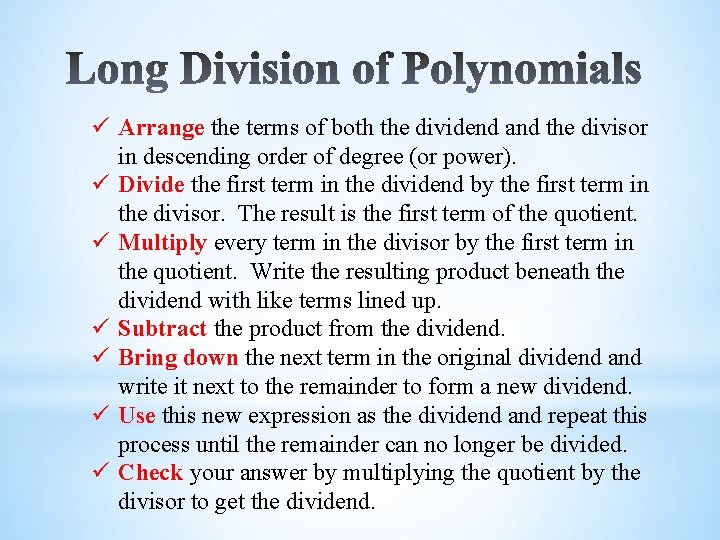Arrange the terms of both the dividend and the divisor in descending order of degree (or power). Divide the first term in the dividend by the first term in the divisor. The result is the first term of the quotient. Multiply every term in the divisor by the first term in the quotient. Write the resulting product beneath the dividend with like terms lined up. Subtract the product from the dividend. Bring down the next term in the original dividend and write it next to the remainder to form a new dividend. Use this new expression as the dividend and repeat this process until the remainder can no longer be divided. Check your answer by multiplying the quotient by the divisor to get the dividend.Using Long Division to Divide a Polynomial Divide using long division. (–y 2 + 2 y 3 + 25) ÷ (y – 3) Step 1: Write the dividend in standard form, including terms with a coefficient of 0. 2 y 3 – y 2 + 0 y + 25 Step 2: Write division in the same way you would when dividing numbers. y – 3 2 y 3 – y 2 + 0 y + 25Step 3 Divide. Notice that y times 2 y 2 is 2 y 3. Write 2 y 2 above 2 y 3. 2 y 2 + 5 y + 15 y – 3 2 y 3 – y 2 + 0 y + 25 –(2 y 3 – 6 y 2) 5 y 2 + 0 y –(5 y 2 – 15 y) 15 y + 25 –(15 y – 45) 70 Multiply 2 y 2 by y – 3. Then subtract. Bring down the next term. Divide 5 y 2 by y. Multiply 5 y by y – 3. Then subtract. Bring down the next term. Divide 15 y by y. Multiply 15 by y – 3. Then subtract. Find the remainder.Step 4 Write the final answer. –y 2 + 2 y 3 + 25 y– 3 = 2 y 2 70 + 5 y + 15 + y – 3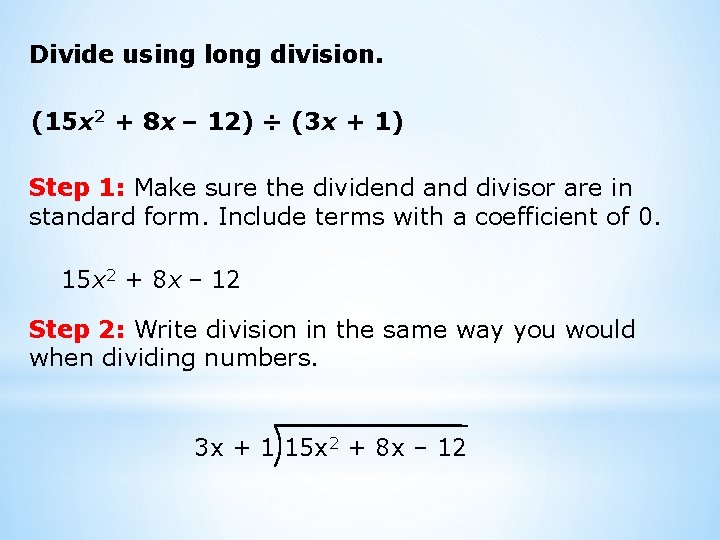Divide using long division. (15 x 2 + 8 x – 12) ÷ (3 x + 1) Step 1: Make sure the dividend and divisor are in standard form. Include terms with a coefficient of 0. 15 x 2 + 8 x – 12 Step 2: Write division in the same way you would when dividing numbers. 3 x + 1 15 x 2 + 8 x – 12Step 3 Divide. Notice that 3 x times 5 x is 15 x 2. Write 5 x above 15 x 2. 5 x + 1 3 x + 1 15 x 2 + 8 x – 12 –(15 x 2 + 5 x) 3 x –(3 x + 1) – 13 Multiply 5 x by 3 x + 1. Then subtract. Bring down the next term. Divide 3 x by 3 x. Multiply 1 by 3 x + 1. Then subtract. Find the remainder.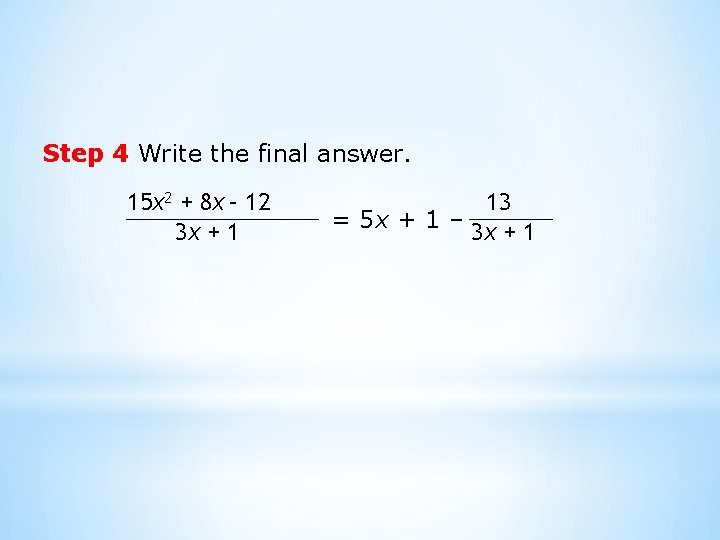Step 4 Write the final answer. 15 x 2 + 8 x – 12 3 x + 1 13 = 5 x + 1 – 3 x + 1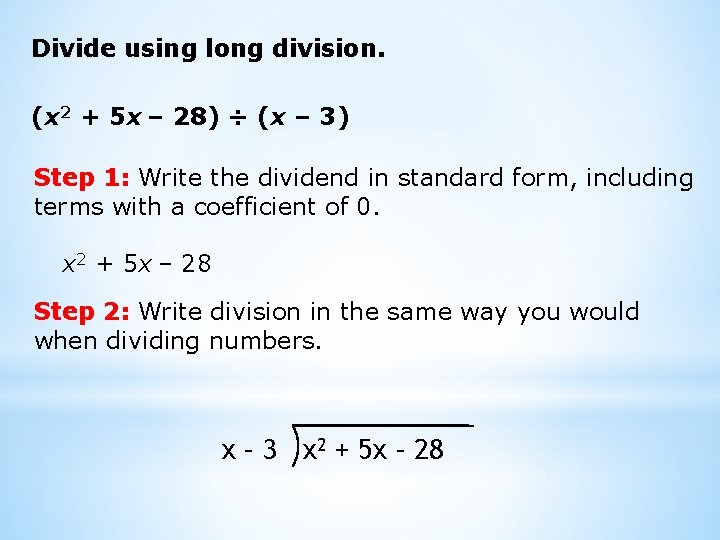Divide using long division. (x 2 + 5 x – 28) ÷ (x – 3) Step 1: Write the dividend in standard form, including terms with a coefficient of 0. x 2 + 5 x – 28 Step 2: Write division in the same way you would when dividing numbers. x – 3 x 2 + 5 x – 28Step 3 Divide. Notice that x times x is x 2. Write x above x 2. x +8 x – 3 x 2 + 5 x – 28 –(x 2 – 3 x) 8 x – 28 –(8 x – 24) – 4 Multiply x by x – 3. Then subtract. Bring down the next term. Divide 8 x by x. Multiply 8 by x – 3. Then subtract. Find the remainder.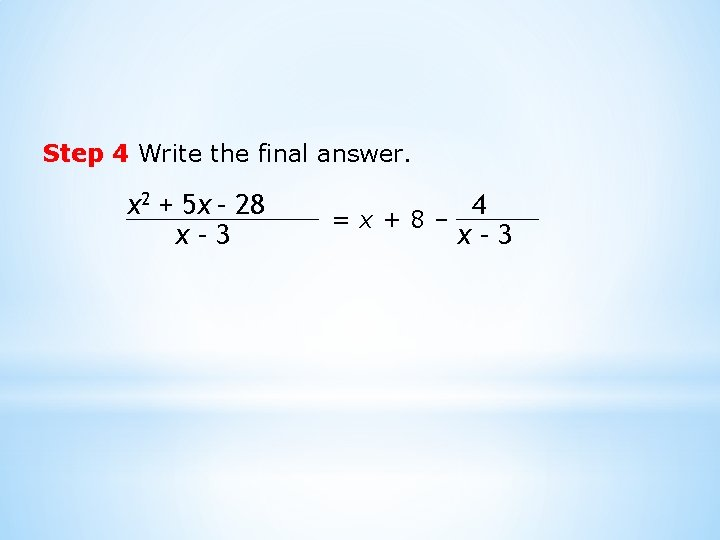Step 4 Write the final answer. x 2 + 5 x – 28 x– 3 4 =x+8– x– 3Using Synthetic Division Synthetic division is a shorthand method of dividing a polynomial by a linear binomial by using only the coefficients. For synthetic division to work, the polynomial must be written in standard form, using 0 as a coefficient for any missing terms as well as a missing constant, and the divisor must be in the form (x – a).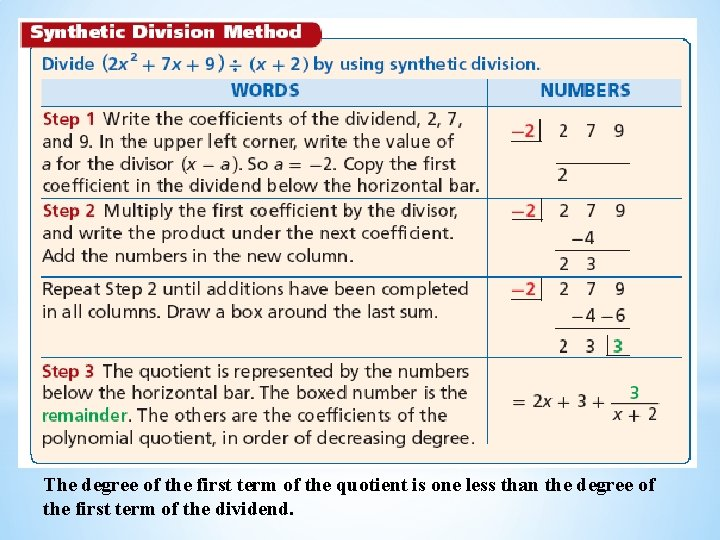The degree of the first term of the quotient is one less than the degree of the first term of the dividend.Divide using synthetic division. (3 x 4 – x 3 + 5 x – 1) ÷ (x + 2) Step 1 Find a. a = – 2 For (x + 2), a = – 2. Step 2 Write the coefficients and a in the synthetic division format. – 2 3– 1 0 5 – 1 Use 0 for the coefficient of x 2.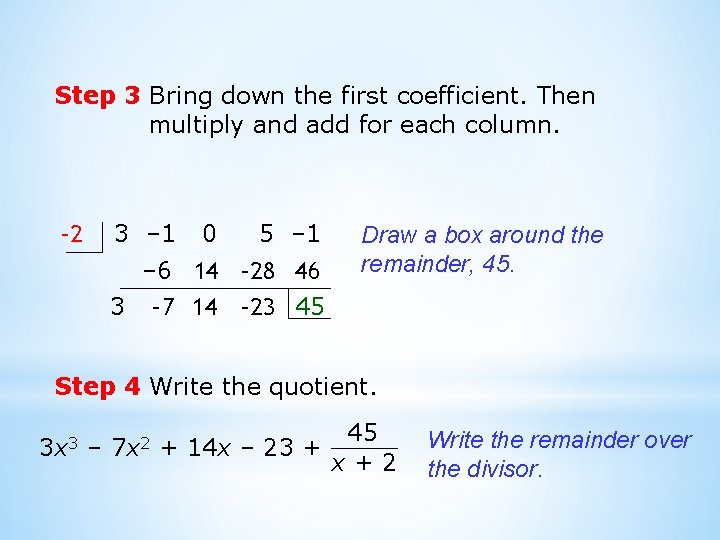Step 3 Bring down the first coefficient. Then multiply and add for each column. – 2 3 – 1 0 5 – 1 – 6 14 – 28 46 3 – 7 14 – 23 45 Draw a box around the remainder, 45. Step 4 Write the quotient. 3 x 3 – 7 x 2 + 14 x – 23 + 45 x+2 Write the remainder over the divisor.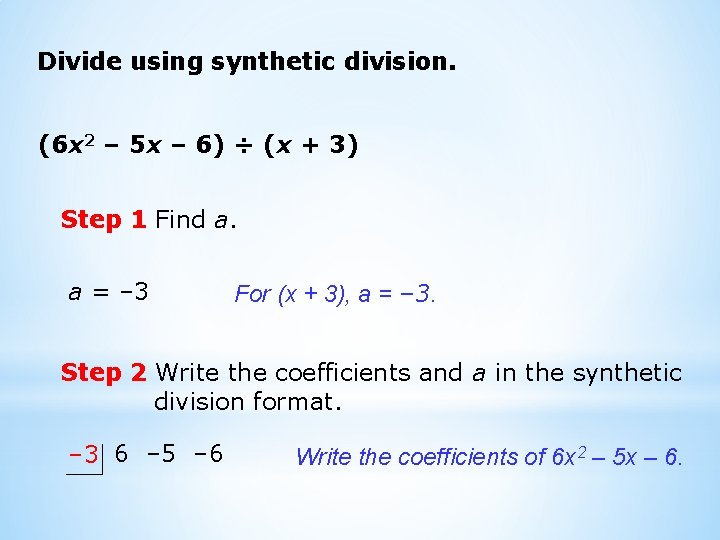Divide using synthetic division. (6 x 2 – 5 x – 6) ÷ (x + 3) Step 1 Find a. a = – 3 For (x + 3), a = – 3. Step 2 Write the coefficients and a in the synthetic division format. – 3 6 – 5 – 6 Write the coefficients of 6 x 2 – 5 x – 6.Step 3 Bring down the first coefficient. Then multiply and add for each column. – 3 6 – 5 – 6 6 – 18 69 – 23 63 Draw a box around the remainder, 63. Step 4 Write the quotient. 6 x – 23 + 63 x+3 Write the remainder over the divisor.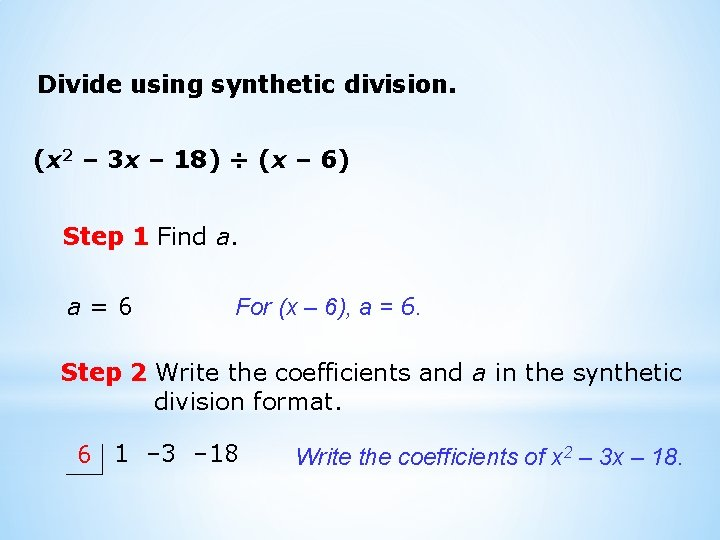Divide using synthetic division. (x 2 – 3 x – 18) ÷ (x – 6) Step 1 Find a. a=6 For (x – 6), a = 6. Step 2 Write the coefficients and a in the synthetic division format. 6 1 – 3 – 18 Write the coefficients of x 2 – 3 x – 18.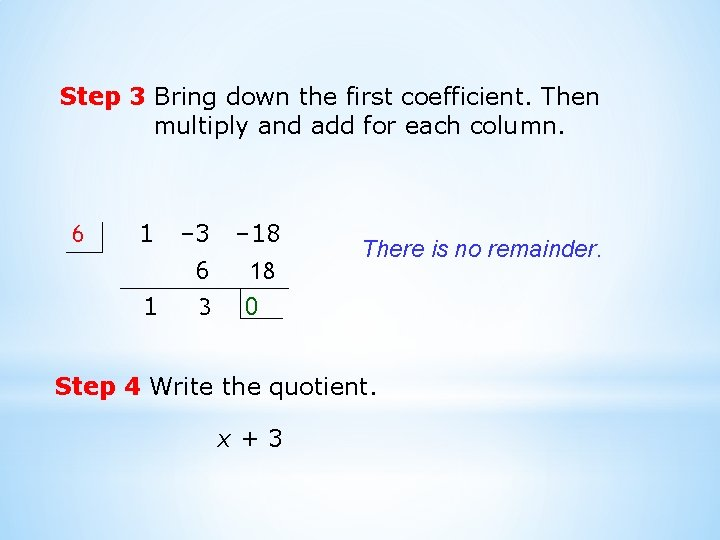Step 3 Bring down the first coefficient. Then multiply and add for each column. 6 1 – 3 – 18 18 1 6 3 There is no remainder. 0 Step 4 Write the quotient. x+3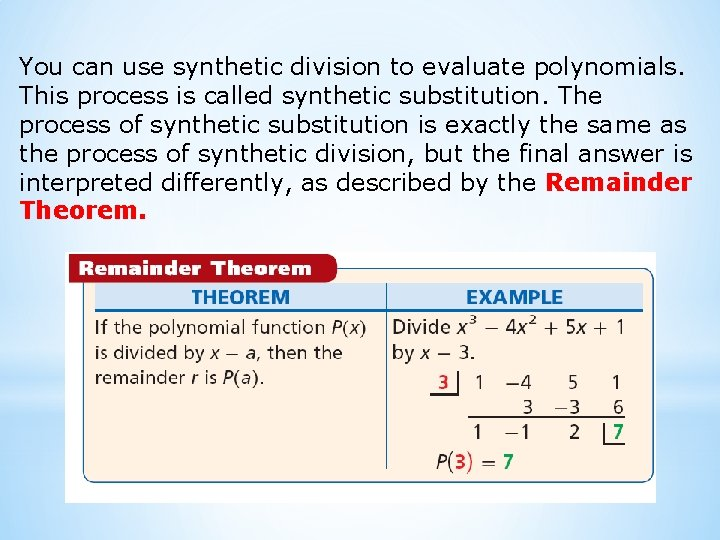You can use synthetic division to evaluate polynomials. This process is called synthetic substitution. The process of synthetic substitution is exactly the same as the process of synthetic division, but the final answer is interpreted differently, as described by the Remainder Theorem.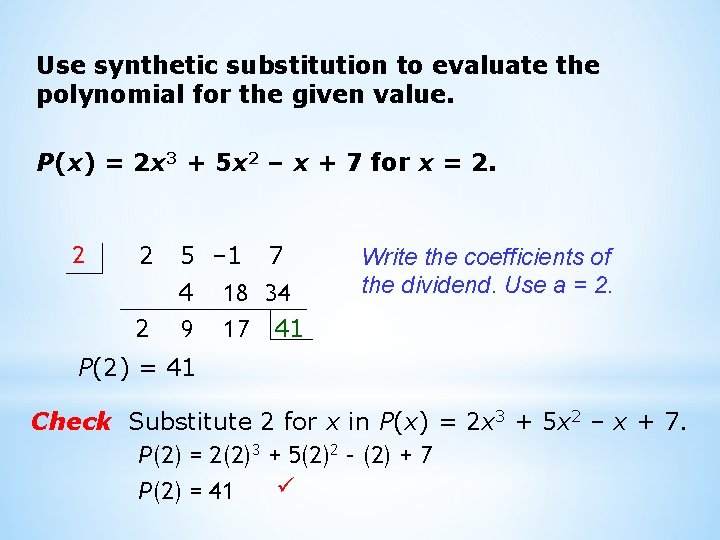Use synthetic substitution to evaluate the polynomial for the given value. P(x) = 2 x 3 + 5 x 2 – x + 7 for x = 2. 2 2 5 – 1 2 4 9 7 18 34 17 41 Write the coefficients of the dividend. Use a = 2. P(2) = 41 Check Substitute 2 for x in P(x) = 2 x 3 + 5 x 2 – x + 7. P(2) = 2(2)3 + 5(2)2 – (2) + 7 P(2) = 41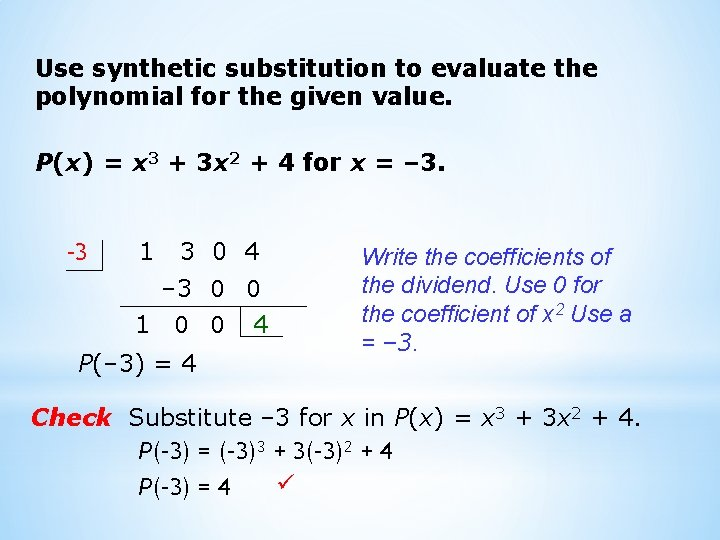Use synthetic substitution to evaluate the polynomial for the given value. P(x) = x 3 + 3 x 2 + 4 for x = – 3 1 3 0 4 – 3 0 0 1 0 0 4 P(– 3) = 4 Write the coefficients of the dividend. Use 0 for the coefficient of x 2 Use a = – 3. Check Substitute – 3 for x in P(x) = x 3 + 3 x 2 + 4. P(– 3) = (– 3)3 + 3(– 3)2 + 4 P(– 3) = 4The Factor Theorem Example:Is this factored completely? No. We have to factor further or use the quadratic formula to solve.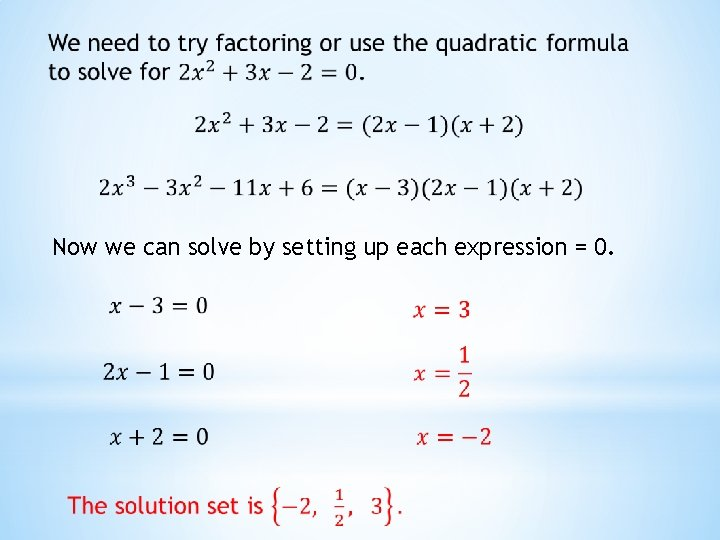Now we can solve by setting up each expression = 0.